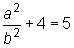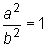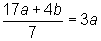Hotline tư vấn: 028.7108 8558 (TP.HCM) / 024.7106 5888 (Hà Nội) / 098 111 3529

# GMAT Question of the Day – 12/12/2020

### QUESTION

If b ≠ 0, does a equal b ?

STATEMENT 1:

a2b2 + 4 = 5

STATEMENT 2:

17a + 4b7 = 3a

(B) Statement (2) BY ITSELF is sufficient to answer the question, but statement (1) by itself is not. (THIS IS CORRECT!)

(C) Statements (1) and (2) TAKEN TOGETHER are sufficient to answer the question, even though NEITHER statement BY ITSELF is sufficient.

We want to determine if there is sufficiency to say that a always equal b or a never equals b.

Statement (1) is the equation. Subtracting 4 from both sides of this equation, we have. Multiplying both sides of this equation by b2, we have a2 = b2. Now having a2 = b2 does not mean that we must have a = b. It is also possible that a = –b. For examples, if a = 4 and b = 4, then a2 = b2, and in this case, a = b, so the answer to the question is “yes.” However, if a = 4 and b = -4, then a2 = a2, and in this case a is not equal to b, so the answer to the question is “no.” Statement (1) is insufficient. We can eliminate choices (A) and (D).

Statement (2) is the equation. Let’s try to rewrite this equation. Multiplying both sides of this equation by 7, we have 17a + 4b = 21a. Subtracting 17a from both sides, we have 4b = 4a. Dividing both sides of this equation by 4, we have b = a. Thus, a = b. We can answer the question with a “yes.” We have determined that a does equal b. Statement (2) is sufficient. Choice (B) is correct.

Tags: ,